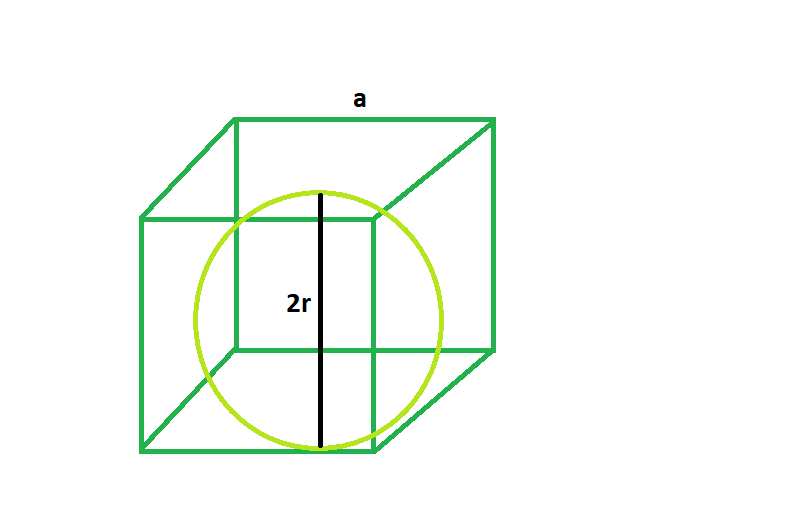# Largest sphere that can be inscribed inside a cube

Given here is a cube of side length a, the task is to find the biggest sphere that can be inscribed within it.
Examples:

```Input: a = 4
Output: 2

Input: a = 5
Output: 2.5```Approach

From the 2d diagram it is clear that, 2r = a
where, a = side of the cube
r = radius of the sphere
so r = a/2.

Below is the implementation of the above approach:

## C++

 `// C++ Program to find the biggest sphere` `// inscribed within a cube` `#include ` `using` `namespace` `std;`   `// Function to find the radius of the sphere` `float` `sphere(``float` `a)` `{`   `    ``// side cannot be negative` `    ``if` `(a < 0)` `        ``return` `-1;`   `    ``// radius of the sphere` `    ``float` `r = a / 2;`   `    ``return` `r;` `}`   `// Driver code` `int` `main()` `{` `    ``float` `a = 5;` `    ``cout << sphere(a) << endl;`   `    ``return` `0;` `}`

## Java

 `// Java Program to find the biggest sphere` `// inscribed within a cube`   `class` `GFG{` `// Function to find the radius of the sphere` `static` `float` `sphere(``float` `a)` `{`   `    ``// side cannot be negative` `    ``if` `(a < ``0``)` `        ``return` `-``1``;`   `    ``// radius of the sphere` `    ``float` `r = a / ``2``;`   `    ``return` `r;` `}`   `// Driver code` `public` `static` `void` `main(String[] args)` `{` `    ``float` `a = ``5``;` `    ``System.out.println(sphere(a));`   `}` `}` `// This code is contributed by mits`

## Python3

 `# Python 3 Program to find the biggest ` `# sphere inscribed within a cube`   `# Function to find the radius ` `# of the sphere` `def` `sphere(a):` `    `  `    ``# side cannot be negative` `    ``if` `(a < ``0``):` `        ``return` `-``1`   `    ``# radius of the sphere` `    ``r ``=` `a ``/` `2`   `    ``return` `r`   `# Driver code` `if` `__name__ ``=``=` `'__main__'``:` `    ``a ``=` `5` `    ``print``(sphere(a))`   `# This code is contributed ` `# by SURENDRA_GANGWAR`

## C#

 `// C# Program to find the biggest ` `// sphere inscribed within a cube ` `using` `System;`   `class` `GFG` `{` `// Function to find the radius ` `// of the sphere ` `static` `float` `sphere(``float` `a) ` `{ `   `    ``// side cannot be negative ` `    ``if` `(a < 0) ` `        ``return` `-1; `   `    ``// radius of the sphere ` `    ``float` `r = a / 2; `   `    ``return` `r; ` `} `   `// Driver code ` `static` `public` `void` `Main ()` `{` `    ``float` `a = 5; ` `    ``Console.WriteLine(sphere(a)); ` `} ` `} `   `// This code is contributed by ajit `

## PHP

 ``

## Javascript

 ``

Output:

`2.5`

Time Complexity: O(1)

Auxiliary Space: O(1)

Feeling lost in the world of random DSA topics, wasting time without progress? It's time for a change! Join our DSA course, where we'll guide you on an exciting journey to master DSA efficiently and on schedule.
Ready to dive in? Explore our Free Demo Content and join our DSA course, trusted by over 100,000 geeks!

Previous
Next